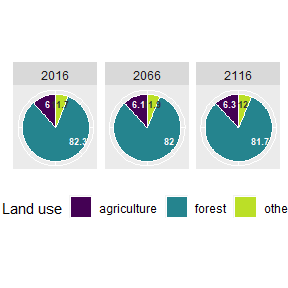# Introduction

European Forestry Dynamics Model (EFDM) is a model for large scale scenario analysis. The model is an area-based matrix model where forest area is stratified with respect to, for example, use, management strategy and development (growth). The model can consider also other land uses, see Scenario 3. In a model run forest area moves in ‘a matrix’ span with the stratifying factors.

The efdm package includes functions to

• define management activities
• estimate transition probabilities
• run an EFDM scenario

and an example dataset for illustration purposes.

In this vignette, we demonstrate ‘efdm’ in running a scenario with the example dataset. Typical workflow in a EFDM scenario analysis starts with preparing the datasets required as an input. Preparing the datasets is usually the most time consuming part of the scenario analysis, and the work flow depends on the data available. Therefore, the preparation is not explained in this vignette. However, the attached datasets offer an example for preparing own datasets.

The efdm is loaded with

library(efdm)

The following packages are used in this vignette to manipulate data and plot results. However, the data manipulation and reporting the results could be done using other packages or even outside the R environment.

library(dplyr)
library(ggplot2)
library(sf)

# Example data

In this vignette we are using example, a collection of example datasets to describe working with efdm. The dataset covers entire Finland and the initial state represents year 2016. However, the dataset is a toy data which is meant for learning the model concept.

data(example)

In this example we have chosen

• a 5-year simulation step
• age and total growing stock volume per hectare to present forest state
• age is classified into 5 year classes, starting from class 1 = 0-5 years, and ending at class 35 = 170+ years with all forests over 170 years old
• volume is classified into 15 classes with class limits depending on the dominant tree species and their growth pattern
• additional stratification variables defining different forest strata are spatial region (North, Middle, South), soil type (Mineral, Peat) and dominant tree species (spruce, other species)

# Activities

In EFDM forest management is represented by activities and activity probabilities. In the first example we have three activities

• thinning (thin)
• final felling (ff)
• no management (noman)

## Activity probability and transition probabilities

The activity probabilities (actprob below) are the probabilities for applying a specific management activity in a specific forest state described by the stratification variables. The activity probabilities are used to allocate forest area to different activities. A valid activity probability specifies activity probabilities for all possible states and activities. All probabilities should be positive and the sum of activity probabilities for each state should be 1.

actprob <- example$actprob actprob #> region soil sp vol age noman thin ff #> 1 South Mineral other 1 1 0.976 0.023 0.001 #> 2 South Mineral other 1 2 0.981 0.019 0.000 #> 3 South Mineral other 1 3 0.994 0.006 0.000 #> 4 South Mineral other 1 4 0.991 0.008 0.001 #> 5 South Mineral other 1 5 0.764 0.024 0.212 #> 6 South Mineral other 1 6 0.974 0.025 0.001 #> [ reached 'max' / getOption("max.print") -- omitted 6294 rows ] Each activity has a transition probability matrix. The transition probability describes movements of forest areas by the simulation time steps. It is used to move a forest state to the next states. A valid transition probability should specify transition probability for each state where the activity can be used. For example if final felling only applies to forests with some minimum volume, then there is no need to specify transitions from states with less than the minimum volume. ## Final felling The final felling activity is represented by a transition matrix that has probability 1 to move any forest to a state where volume and age are in the smallest class. The target state is independent of the starting state. It will always be vol=1 and age=1, the smallest class in the stratification. transprobs_ff <- expand.grid(vol0=1:15, age0=1:35, vol1=1, age1=1, prob=1) transprobs_ff #> vol0 age0 vol1 age1 prob #> 1 1 1 1 1 1 #> 2 2 1 1 1 1 #> 3 3 1 1 1 1 #> 4 4 1 1 1 1 #> 5 5 1 1 1 1 #> 6 6 1 1 1 1 #> 7 7 1 1 1 1 #> 8 8 1 1 1 1 #> 9 9 1 1 1 1 #> 10 10 1 1 1 1 #> [ reached 'max' / getOption("max.print") -- omitted 515 rows ] In efdm the final felling activity is defined by giving • name of the activity, “ff” • names of the dynamic variables, that is the variables that the final felling activity affects, “vol” and “age” • transition probabilities ff <- define_activity("ff", c("vol", "age"), transprobs_ff) ## No management If there are no management activities or treatments in the forest, “no management” activity is used to make the forest grow. In this example, we assume that growth changes the values of volume and age and is dependent on region, soil type and tree species. Moreover, we assume that the growth is so different in between regions and tree species, that it should be estimates completely separately for each class. ### Estimation of transition matrix using pair data To estimate the transition matrix, we use “pair data” obtained from two consecutive measurements of permanent sample plots in the national forest inventory. For estimatetransprobs, the estimation function implemented in efdm, the pair data is a data.frame of pairs of dynamic variables and possible stratification variables, which affect the growth but are not affected by growth. example$noman_pairs
#>   region    soil     sp vol0 vol1 age0 age1
#> 1  South Mineral spruce   10    7   13   14
#> 2  South Mineral spruce    8    8   20   21
#> 3  South Mineral  other    9   10    5    6
#> 4  South Mineral  other    1    1    1    2
#> 5  South Mineral  other    7    8    2    3
#> 6  South Mineral  other    7   10    5    6
#> 7  South Mineral  other    3    5   31   32
#>  [ reached 'max' / getOption("max.print") -- omitted 15535 rows ]

In order to use estimatetransprobs we still need two parts, a state space and a prior. The state space and prior are required because the pair data might not have observations for all possible states, but the transition matrix should be available for all states.

#### State space

The state space is the collection of all possible states of stratifying variables. Since we have an activity probability for each state we can use the actprob to obtain the statespace

statespace <- actprob %>% select(c(region, soil, sp, vol, age))
statespace
#>    region    soil    sp vol age
#> 1   South Mineral other   1   1
#> 2   South Mineral other   1   2
#> 3   South Mineral other   1   3
#> 4   South Mineral other   1   4
#> 5   South Mineral other   1   5
#> 6   South Mineral other   1   6
#> 7   South Mineral other   1   7
#> 8   South Mineral other   1   8
#> 9   South Mineral other   1   9
#> 10  South Mineral other   1  10
#>  [ reached 'max' / getOption("max.print") -- omitted 6290 rows ]

In this example dataset the number of states in the state space is the product of the number of classes in each stratifying variable, that is $$3*2*2*15*35 = 6300$$.

#### Prior

The prior in estimatetransprobs works by adding one observation to the pair data for each starting state. The efdm has a few standard prior choices.

• prior_grow(variable) grows variable by one class.
• "nochange", forest state is not changed
• "uninformative", an observation with tiny weight is added to every possible target state.

For the no management activity we are using prior_grow("age") stating that there is always at least one observation where the age grows by one class but the volume is not changing.

#### Estimation

Now we are ready to estimate the transition probabilities for no management

transprobs_noman <- estimatetransprobs(c("vol", "age"), # the dynamic variables of the activity
example$noman_pairs, # pair data for no management statespace=statespace, factors=c("soil"), # Information for different soil types # is used with smaller weight by=c("region","sp"), # Separate estimation for each # region and species prior=prior_grow("age")) and to define the activity noman noman <- define_activity("noman", c("vol", "age"), transprobs_noman) The resulting transition probabilities look like transprobs_noman %>% arrange(vol0, age0, soil, region, sp, vol1, age1) #> vol0 age0 soil region sp vol1 age1 prob #> 1 1 1 Mineral Middle other 1 2 0.629107981 #> 2 1 1 Mineral Middle other 2 2 0.145539906 #> 3 1 1 Mineral Middle other 3 2 0.122065728 #> 4 1 1 Mineral Middle other 4 2 0.089201878 #> 5 1 1 Mineral Middle other 5 2 0.009389671 #> 6 1 1 Mineral Middle other 6 2 0.004694836 #> [ reached 'max' / getOption("max.print") -- omitted 13180 rows ] ## Thinning We use exactly the same procedure for thinning that we used for no management. The only differences are the activity name and the pair data used. transprobs_thin <- estimatetransprobs(c("vol", "age"), example$thin_pairs, # pair data for thinning
statespace=statespace,
factors=c("soil"),
by=c("region","sp"),
prior=prior_grow("age"))
thin <- define_activity("thin", c("vol", "age"), transprobs_thin)

# Scenario 1

EFDM is an area based model. The initial state of the scenario is a data.frame where for each state there is an area (zeros may be omitted)

state0 <- example$initial_state state0 #> region soil sp vol age area #> 1 South Mineral other 1 1 73518.749 #> 2 Middle Mineral other 1 1 105532.353 #> 3 North Mineral other 1 1 49583.432 #> 4 South Peat other 1 1 15035.772 #> 5 Middle Peat other 1 1 33996.388 #> 6 North Peat other 1 1 2039.203 #> 7 South Mineral spruce 1 1 186533.582 #> 8 Middle Mineral spruce 1 1 63984.635 #> [ reached 'max' / getOption("max.print") -- omitted 3271 rows ] Next, we run the EFDM scenario for 20 time steps (100 years) starting with initial state state0, activity probabilities actprob and a list of activities. activities <- list(noman, thin, ff) states1 <- runEFDM(state0, actprob, activities, 20) runEFDM produces a data.frame of areas allocated to each activity at each time step. states1 %>% arrange(soil, region, sp, vol, age, time, activity) #> soil region sp vol age area activity time #> 1 Mineral Middle other 1 1 105.53235 ff 0 #> 2 Mineral Middle other 1 1 101627.65637 noman 0 #> 3 Mineral Middle other 1 1 3799.16472 thin 0 #> 4 Mineral Middle other 1 1 99.11577 ff 1 #> 5 Mineral Middle other 1 1 95448.48821 noman 1 #> 6 Mineral Middle other 1 1 3568.16778 thin 1 #> [ reached 'max' / getOption("max.print") -- omitted 209007 rows ] ## Analysing results ### Result coefficients Using so called result coefficients is a way to obtain results with respect to other forest properties than area. In this scenario we have growing stock volume, drain and harvest income as result variables. The volume coefficients convert volume class vol and dominant species sp into growing stock volume volume (m3/ha) in four tree species groups (species): pine, spruce, broadleaves and all. The volume coefficients were estimated for the classes based on the species composition in the forest inventory data as class averages. example$vol_coef %>%
arrange(vol, sp, species)
#>    vol     sp   species     volume
#> 1    1  other       all 0.50632300
#> 2    1  other broadleaf 0.12501893
#> 3    1  other      pine 0.30152225
#> 4    1  other    spruce 0.07978181
#> 5    1 spruce       all 1.20000000
#> 6    1 spruce broadleaf 0.37598659
#> 7    1 spruce      pine 0.09533751
#> 8    1 spruce    spruce 0.72867590
#> 9    2  other       all 3.39429400
#> 10   2  other broadleaf 0.74911648
#> 11   2  other      pine 2.16464343
#> 12   2  other    spruce 0.48053409
#>  [ reached 'max' / getOption("max.print") -- omitted 108 rows ]

Drain is the harvest accumulation which is linked to management activities (activity), and separated by the volume class (vol) and dominant species (sp). It was also estimated from the observed changes in the forest inventory data as class averages. The drain is given as m3 per ha (drain) and timber assortment (assort): pulp/saw wood.

example$drain_coef %>% arrange(-vol, sp, assort, activity) #> vol sp drain assort activity #> 1 15 other 203.57043 pulp ff #> 2 15 other 61.07113 pulp thin #> 3 15 other 197.54969 saw ff #> 4 15 other 59.26491 saw thin #> 5 15 spruce 165.51780 pulp ff #> 6 15 spruce 49.65534 pulp thin #> 7 15 spruce 318.13899 saw ff #> 8 15 spruce 95.44170 saw thin #> 9 14 other 172.94581 pulp ff #> 10 14 other 51.88374 pulp thin #> [ reached 'max' / getOption("max.print") -- omitted 110 rows ] Income is loosely based on the actual statistics of Finnish timber assortment prices over last years in unit eur/m3. example$income_coef
#>   assort activity euro
#> 1    saw       ff   56
#> 2    saw     thin   44
#> 3   pulp       ff   20
#> 4   pulp     thin   15

### Results

Simulation timesteps are mutated to mid-years of simulation steps (the simulation begins from year 2016):

states1 <- states1 %>% mutate(time = factor(2016 + time*5))

Growing stock volume is estimated by merging the area distribution by simulation steps (states1) and volume coefficients. The total growing stock volume (m3) is a result of multiplication of area (ha) and coefficient (m3/ha). Finally the result is visualized in a figure.

volume <- merge(states1, example$vol_coef) %>% mutate (volume=area*volume) %>% #area is multiplied with m3/ha volume the get total volume in m3 filter(species != "all") ggplot(volume) + scale_fill_viridis_d(end = 0.9) + geom_bar(aes(x=time, weight=volume/1000000000, fill=species)) + labs(y=NULL,title=expression(paste("Growing stock, bil.",m^3)), x="Year", fill="") + theme(legend.position = "bottom", axis.text.x = element_text(angle = -90))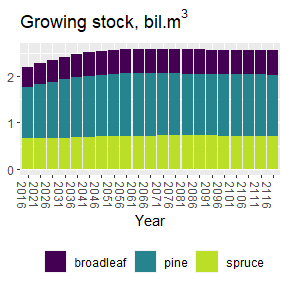Estimating age distribution does not require result coefficients. However, the age classes are defined for 50-year classes instead of original 5-year classes, and then plotted for only three time steps: years 2016, 2066 and 2116. states1$ageclass <- cut(states1$age, breaks=c(0,10,20,30,35), include.lowest = TRUE, #labels=c("0-50","51-100","101-150","150+")) labels=c("-50","-100","-150","150+")) states1$region <- factor(states1$region, labels = c("South", "Middle", "North")) ggplot(subset(states1, time %in% c(2016,2066,2116))) + geom_bar(aes(x=ageclass, weight=area/1000000, fill=region)) + scale_fill_viridis_d(end = 0.9) + facet_grid(cols=vars(time)) + labs(y=NULL, title="Area, mill.ha", x="Ageclass", fill=NULL) + theme(legend.position = "bottom", axis.text.x = element_text(angle = -90))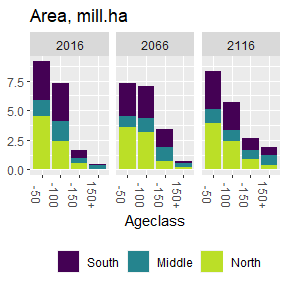Income is estimated by first converting the drain (m3/ha) into income (eur/ha). Then multiplication with area (ha) gives the euros. euro <- merge(example$drain_coef, example$income_coef) %>% mutate(euro = euro*drain) removal <- merge(states1, euro) %>% mutate(income=euro*area) ggplot(subset(removal,!time %in% c(2116))) + geom_bar(aes(x=time, weight=income/5000000000, fill=assort)) + scale_fill_viridis_d(end = 0.9) + labs(y=NULL,title = "Income, bil.€/year", x="5-year intervals", fill=NULL ) + theme(legend.position = "bottom", axis.text.x = element_text(angle = -90))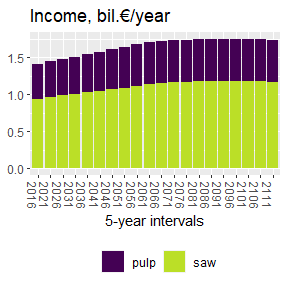# Scenario 2 In this example the tree species changes after final felling. Therefore we redefine final felling activity taking into account in addition to volume and age also the dominant species. The change probability depends on region and dominant species. Volume and age act as before. They move to the smallest classes (vol1=1 and age1=1). transprobs_ff_age_species <- statespace %>% select(vol0=vol, age0=age, sp0=sp, region) %>% unique %>% group_by(region, sp0, vol0, age0) %>% summarize(data.frame(vol1=1, age1=1, sp1=c('other','spruce'), prob=case_when( sp0=='other' && region=='South' ~ c(1, 0), sp0=='other' && region=='Middle' ~ c(1, 0), sp0=='other' && region=='North' ~ c(0.8, 0.2), #in other dominated forest # in northern boreal vegetation zone 20% of final felled area # change to spruce dominated forest sp0=='spruce' && region=='South' ~ c(0.3, 0.7), sp0=='spruce' && region=='Middle' ~ c(0.2, 0.8), sp0=='spruce' && region=='North' ~ c(0, 1)))) #> summarise() has grouped output by 'region', 'sp0', 'vol0', 'age0'. You can override using the .groups argument. ff_age_species <- define_activity("ff", c("vol", "age", "sp"), transprobs_ff_age_species) The list of activities includes the same noman and thin as in Scenario 1, and the above defined final felling. The same initial state as in Scenario 1 is run with the new list of activities and stored in variable states2. activities2 <- list(noman, thin, ff_age_species) states2 <- runEFDM(state0, actprob, activities2, 20) The proportion of spruce dominated forest area is estimated for time steps 0, 10 and 20 (years 2016, 2066 and 2116). And the result is presented by the vegetation zones as a map. # Compute proportion of spruces in each time and region prop_spruces <- states2 %>% group_by(region, time) %>% summarise(proportion = 100*sum((sp=="spruce")*area)/sum(area)) %>% filter(time %in% c(0, 10, 20)) %>% mutate(time = 2016 + 5*time) #> summarise() has grouped output by 'region'. You can override using the .groups argument. # Add bio-geographical regions (MetsaKasvVyoh provided in efdm package) to the data prop_spruces <- merge(MetsaKasvVyoh, prop_spruces) prop_spruces %>% ggplot() + geom_sf(aes(fill=proportion)) + facet_wrap(vars(time)) + scale_fill_gradient(low = "white", high = "forestgreen") + labs(fill="Proportion" ,title="Proportion of spruce dominated forests, %") + guides(fill = guide_colourbar(frame.colour = "grey20",ticks.colour = "grey20")) + theme(legend.position = "bottom", axis.text.x = element_text(angle = -90))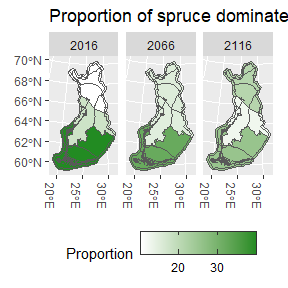The map provided by: Finnish Environment Institute # Scenario 3 - Land use changes First we add two land use classes to the statespace. Agriculture is stratified according to region and soil type, while other land use is only stratified according to region. statespace3 <- statespace statespace3$landuse <- "forest"
agriculture <- unique(statespace3 %>% mutate(sp=0, vol=0, age=0, landuse="agriculture"))
other <- unique(statespace3 %>% mutate(soil=0, sp=0, vol=0, age=0, landuse="other"))
statespace3 <- rbind(statespace3, agriculture, other)

We use separate activities for deforestation to each land use class. Variables (vol, age, sp) not used by the agriculture are set to 0. Soil type and region are not changing as a result of deforestation to agriculture.

transprobs_defor_to_agri <- statespace3 %>%
filter(landuse=="forest") %>%
select(vol0=vol, age0=age, sp0=sp,landuse0=landuse) %>%
unique %>%
mutate(vol1=0, age1=0, sp1=0, landuse1="agriculture", prob=1)
defor_to_agri <- define_activity("defor_to_agri",
c("vol", "age", "sp", "landuse"),
transprobs_defor_to_agri)

Deforestation to other land use also changes soil type to 0.


transprobs_defor_to_other <- statespace3 %>%
filter(landuse=="forest") %>%
select(soil0=soil, vol0=vol, age0=age, sp0=sp,landuse0=landuse) %>%
unique %>%
mutate(soil1=0, vol1=0, age1=0, sp1=0, landuse1="other", prob=1)
defor_to_other <- define_activity("defor_to_other",
c("soil", "vol", "age", "sp", "landuse"),
transprobs_defor_to_other)

Afforestation only applies to agriculture. Volume and age classes start from 1 and the area is split evenly to spruce and other species.

transprobs_aff <- statespace3 %>%
filter(landuse=="agriculture") %>%
select(soil, vol0=vol, age0=age, region, sp0=sp, landuse0=landuse) %>%
mutate(vol1=1, age1=1, sp1="other", landuse1="forest", prob=1)
transprobs_aff <- rbind(transprobs_aff %>% mutate(sp1="spruce", prob=0.5),
transprobs_aff %>% mutate(sp1="other", prob=0.5))
affor <- define_activity("affor",
c("vol", "age", "sp", "landuse"),
transprobs_aff)

A donothing activity is used for non-forest land uses, when there is nothing forestry related going on.

donothing <- define_activity("donothing", character())
activities3 <- list(noman, thin, ff, defor_to_other, defor_to_agri, affor, donothing)

adding land use information to state

state03 <- state0
state03$landuse <- "forest" state03 <- rbind(state03, agriculture %>% mutate(area=rep(c(1552000/2,997000/2,76000/2),each=2)), other %>% mutate(area=c(721000,507000,112000))) Activity probabilities for new land uses actprob3 <- actprob actprob3$landuse <- "forest"
# Define probabilities for new activities
actprob3$defor_to_other <- 0.0002 actprob3$defor_to_agri <- 0.00025
actprob3$affor <- 0 actprob3$donothing <- 0
# Normalise probabilities after adding new activities
actnames <- c("noman", "thin", "ff", "defor_to_other", "defor_to_agri", "affor", "donothing")
actprob3[actnames] <- actprob3[actnames]/rowSums(actprob3[actnames])
# Define activity probabilities also for agriculture and other land uses
actprob3 <- rbind(actprob3,
agriculture %>% mutate(thin=0, ff=0, noman=0, defor_to_other=0, defor_to_agri=0, affor=0.0005, donothing=0.9995),
other %>% mutate(thin=0, ff=0, noman=0, defor_to_other=0, defor_to_agri=0, affor=0, donothing=1))
states3 <- runEFDM(state03, actprob3, activities3, 20)
#pie charts of land use areas
LUareas <- states3 %>%
group_by(time, landuse) %>%
summarise(area = sum(area)) %>%
ungroup()
#> summarise() has grouped output by 'time'. You can override using the .groups argument.
LUareas <- LUareas %>%
mutate(time = factor(time, labels = seq(2016,2116,5)))
totalarea <- sum(state03\$area)
LUareas %>% filter(time %in% c("2016","2066","2116")) %>%
mutate(area = area/totalarea) %>%
ggplot() +
geom_bar(aes(x="",y=area, fill=landuse), stat="identity", width=1, color = "white") +
scale_fill_viridis_d(end = 0.9) +
coord_polar("y", start=0) +
facet_wrap(vars(time)) +
labs(y=NULL,fill="Land use", x=NULL)+
theme(axis.text.x = element_blank(),
axis.ticks = element_blank()) +
geom_text(aes(x="",y = rep(c(0.03, 0.33, 0.95), 3), label = round(area*100,1)),
nudge_x=0.25, size=2.25,
color=rep(c("grey20","white","white"), 3), fontface="bold") +
theme(legend.position = "bottom")